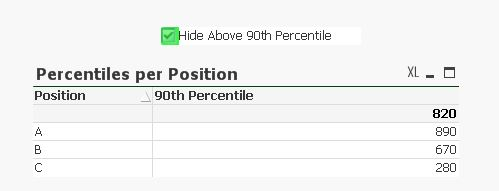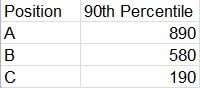# QlikView App Development

Discussion Board for collaboration related to QlikView App Development.

Highlighted
New Contributor III

## Calculation of Percentiles (fractile within fractile)

My data set is:

IDPositionSalary
1C100
2C200
3C300
4B400
5B500
6B600
7B700
8A800
9A900
10A1000

My first task is to calculate 90th percentile per Position. That I can achieve easy with expression Fractile(Salary, .9)My second task is what got me totally stuck and out of ideasI have to implement ability for user to exclude salaries above or equal 90th percentile and to recalculate 90th percentile again...per position.

So what I have achieved is:

1. Created load script

HideAbove90thPercentile:

[HideAbove90thPercentile

"Hide Above 90th Percentile"

];

2. Created a listbox with checkbox type:3.Created variable v90:

=Fractile(Salary, .9)

4. In my straight table Percentile per Position created expression:

=if(GetSelectedCount([HideAbove90thPercentile])=0,

Fractile(Salary, .9),

if(GetSelectedCount([HideAbove90thPercentile])=1,

Fractile({\$<Salary ={"<=\$(v90)"}>}Salary, .9)

))

So the result I get is incorrect. Variable v90 gets calculated before chart and it's value is for all the positions. So salary in the expression above is compared not by 90th percentile per position, but whole set of positions. Is it possible to calculate it per row level/single position? That it would show correct 90th Percentile per position in the chart below. Now it shows incorrect for B and C. We can see correct values only if we select single positions.

Workaround to create variables for each of positions wouldn't work since in a real life application I have hundreds of different positions.

Result:Should be:Please see the file attached.

Tags (4)
1 Solution

Accepted SolutionsMVP

## Re: Calculation of Percentiles (fractile within fractile)

You probably need to use advanced aggregation here to include your Fractile() calculation into another Fractile():

=if(GetSelectedCount([HideAbove90thPercentile])=0,

Fractile(Salary, .9),

if(GetSelectedCount([HideAbove90thPercentile])=1,

Fractile(Aggr( If( Salary < Fractile(TOTAL<Position> Salary,.9),Salary),Position, ID) ,.9)

))

2 RepliesMVP

## Re: Calculation of Percentiles (fractile within fractile)

You probably need to use advanced aggregation here to include your Fractile() calculation into another Fractile():

=if(GetSelectedCount([HideAbove90thPercentile])=0,

Fractile(Salary, .9),

if(GetSelectedCount([HideAbove90thPercentile])=1,

Fractile(Aggr( If( Salary < Fractile(TOTAL<Position> Salary,.9),Salary),Position, ID) ,.9)

))

New Contributor III

## Re: Calculation of Percentiles (fractile within fractile)

Yes, that works perfectly. Thanks for the help!